# Sine-Gordon equation

(diff) ← Older revision | Latest revision (diff) | Newer revision → (diff)

A relativistically-invariant equation in two space-time variables, of the form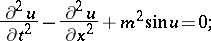(1)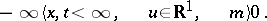The name was suggested by M. Kruskal, in analogy with the linear Klein–Gordon equation (whereappears in place of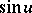). In characteristic (light-cone) variables the sine-Gordon equation has the form(2)

In both cases (1) and (2), the sine-Gordon equation admits a Lax representationwhereandare linear operators and. This enables one to obtain solutions of the Cauchy problem by using the inverse scattering method.

The Cauchy problem for the sine-Gordon equation is formulated in the following way.

Case (1):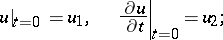Case (2):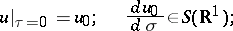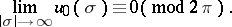Here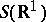is the Schwartz space of rapidly-decreasing functions. Under certain additional restrictions on the initial conditions, the Cauchy problems (1) and (2) can be solved uniquely, and their solution sets coincide. The evolution of the scattering data for the corresponding-operators is given by explicit formulas, and solutionsandcan be found by using integral equations of Gel'fand–Levitan–Marchenko type.

The periodic problem for the sine-Gordon equation can be studied by means of an algebraic-geometric method (similar to the case of the Korteweg–de Vries equation). In particular, one obtains explicit expressions for the finite-gap solutions of the sine-Gordon equation in terms of-functions on the corresponding Abelian varieties.

The Hamiltonian version of the sine-Gordon equation (in case (1), for example) is the Hamiltonian system with Hamiltonian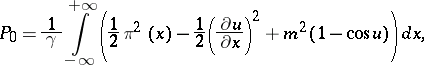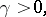and symplectic form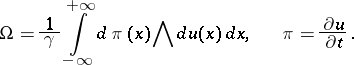This system is completely integrable, and replacing the variablesandby the scattering data of the corresponding operatoryields a canonical transformation into variables of action-angle type. The phase space is parametrized by canonically adjoint variables of three types:

1)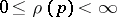,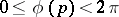,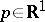;

2)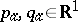,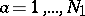,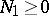,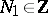;

3)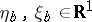,,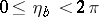,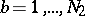,,.

The total energy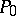and total momentumof the fieldin terms of the new variables are as follows: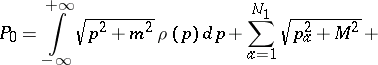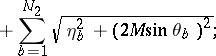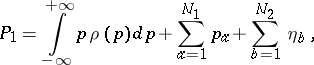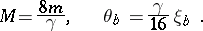In case (1) one also obtains a completely-integrable Hamiltonian system.

## An application to quantum field theory.

Letbe a scalar field with Lagrangian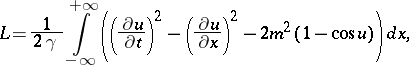whereis the constant of interaction. The sine-Gordon equation is the Euler–Lagrange equation for this Lagrangian. In the quasi-classical quantization of the field, a fundamental role is played by the above formulas forand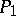. The first terms on their right-hand sides correspond to particles of massand to particles of the ground field, respectively (cf. also Soliton). The second and third terms correspond to localized solutions of the sine-Gordon equation, namely solitons and dual solitons with massesand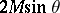, respectively. The system obeys the conservation law (of topological charge):Particles of the first and third types have charge 0, while those of the second type have charge. Particles with equal charges repulse, while those with different charges attract. The presence of infinitely-many conservation laws means that the number of particles of each type is preserved under scattering; the-particle-matrix reduces to paired-matrices (cf. Scattering matrix). Using integrals over trajectories (cf. Integral over trajectories), one can compute the quantum corrections to the masses and to the quasi-classical-matrix of solitons. One of the non-trivial properties of the above model is the appearance of a whole spectrum of particles (solitons), while the Lagrangian of the theory contains only one field. Furthermore, in the weak interaction approximation (that is, whenis small), solitons are heavy particles and interact strongly.

How to Cite This Entry:
Sine-Gordon equation. Encyclopedia of Mathematics. URL: http://encyclopediaofmath.org/index.php?title=Sine-Gordon_equation&oldid=18822
This article was adapted from an original article by L.A. Takhtadzhyan (originator), which appeared in Encyclopedia of Mathematics - ISBN 1402006098. See original article